Three-Level Direct Torque Control Based on Artificial Neural Network of Double Star Synchronous Machine

Elakhdar BENYOUSSEF1, Abdelkader MEROUFEL1 and Said BARKAT2

1 Faculty of Science and Engineering, Department of Electrical Engineering, University of Djilali Liabes, Sidi Bel Abbes 22000, BP 89 Algeria

2 Faculty of Science and Engineering, Department of Electrical Engineering, M’sila University, Ichbilia Street, M’sila 28000, Algeria

E-mails: lakhdarbenyoussef@yahoo.com; ameroufel@yahoo.fr; Sa_barkati@yahoo.fr

Abstract

This paper presents a direct torque control strategy for double star synchronous machine fed by two three-level inverters. The analysis of the torque and the stator flux linkage reference frame shows that the concept of direct torque control can be extended easily to double star synchronous machine. The proposed approach consists to replace the switching tables by one artificial neural networks controller. The output switching states vectors of the artificial neural networks controller are used to control the two three-level inverters. Simulations results are given to show the effectiveness and the robustness of the suggested control method.

Keywords

Double Star Synchronous Machine; Multi-Level Inverter; Direct Torque Control; Artificial Neural Networks Control.

Introduction

Today, three-phase machines are a standard for electrical drives . Cost, reliability, robustness, and maintenance-free operation are among the main reasons behind the penetration of this kind of machines in industrial applications . Also, the development of power electronics and signal processing has resulted in the improvement of the control for AC drives. However, the limited power supply levels, the system performance requirements motivate the use of more than three phases .

In a multiphase drive, here assumed to be a drive that comprises more than the conventional three phases, the machine output power can be divided into two or more solid state inverters that could each be kept within prescribed power limits. Also, having additional phases to control means additional degrees of freedom available for further improvements in the drive system. Major advantages of using a multiphase machine instead of a three-phase machine are higher torque density, greater efficiency, reduced torque pulsations, greater fault tolerance and reduction in the required rating per inverter leg (and therefore simpler and more reliable power-conditioning equipment) . Additionally, noise characteristics of the drive improve as well .

One of the most common examples of multiphase machines is the double star synchronous machine (DSSM). Such a machine has the advantage of reducing the electromagnetic torque ripples and rotor losses significantly . To introduce an electric motor in high power applications, such as traction or marine propulsion, it is often necessary to segment the power . To this end, one can intervene at the converter level through multilevel or parallel topologies.

Among the multilevel inverters generally quoted in the literature, the three-level neutral-point-clamped (NPC) inverter has the advantage of being able to produce three different levels of output phase voltage compared to the standard two-level one . With a greater number of available levels for the output voltage the desired sinusoidal voltage can be achieved more accurately without increasing the switching frequency; making possible to use less expensive semiconductor devices. This results in less current harmonics and therefore more efficient utilization of the available energy. However, this improvement is at the expense of additional hardware and complexity .

During the last two decades, the control of electrical drives has been the subject of intensive investigations. Especially, direct torque control (DTC) is recognized today as a high-performance control strategy for AC drives. The conventional DTC is characterized by its good dynamic performances and robustness, because it requires no current regulator, no coordinate transformation and depends only on stator resistance . Considerable research effort is still being devoted to the elimination of its inherent disadvantages. One more significant disadvantage of conventional DTC is torque and flux ripples. Recently, several techniques have been developed [1, 3] to improve the torque performance. In this context, the direct torque control using artificial neural networks (DTC-ANN) attracts more and more the attention of many scientists [9, 10]. This control method exploits the ability of the artificial neural network to approximate nonlinear functional relationships.

This paper is devoted to DTC-ANN of DSSM fed by two three-level NPC inverters. This control scheme combines the advantages of multilevel inverters and artificial neural networks for decoupled flux and torque control.

Modelling of the Double Star Synchronous Machine

For idealized DSSM the following equations of the instantaneous stator phase voltages can be written:(1)

where vs1, vs2: Stator voltages; is1, is2: Stator currents; фs1, фs2: Stator flux; Ф: Flux of rotor excitation, vf, if: Voltage and current of rotor excitation.

The original six dimensional system of the machine can be decomposed into three orthogonal subspaces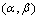,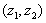and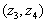, using the following transformation.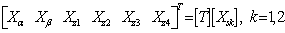(2)

where: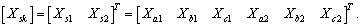,

(Xsk) represents stator currents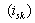, stator flux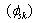, or stator voltages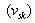.(3)

To express the stator and rotor equations in the same reference frame, the following rotation transformation is adopted.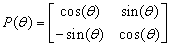(4)

With: θ is the rotor position and y is set to π/6.

With this transformation, the components of the α-β plane can be expressed in the d-q plane:

The electrical equations(5)

where vd, vq: Stator voltages dq components; id, iq: Stator currents dq components; фd, фq : Stator flux dq components.

The flux equations(6)

The mechanical equation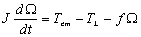(7)

where Tem, TL: Electromagnetic and load torque; : Rotor speed; J: Moment of inertia; f: Friction coefficient.

The electromagnetic torque is given by: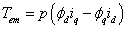(8)

where p: Number of pairs of poles; id, iq: Stator currents dq components; фd, фq : Stator flux dq components.

Structure of the Three-Level Inverter

A three-level inverter differs from a conventional two-level inverter in that it is capable of producing three different levels of output phase voltage. The structure of a three-level neutral point clamped inverter is shown in Figure 1. When switches 1 and 2 are on the output is connected to the positive supply rail. When switches 3 and 4 are on, the output is connected to the negative supply rail. When switches 2 and 3 are on, the output is connected to the supply neutral point via one of the two clamping diodes .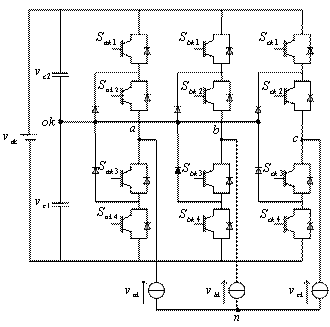Figure 1. Schematic diagram of a three-level NPC inverter (k=1 for first inverter and k=2 for second inverter)

Table 1 shows the switching states of three-level NPC inverter. Since three kinds of switching states exist in each phase, a three-level inverter has 27 switching states.

Table 1. Switching states of a three-level inverter (with x=a, b, c)

 Switching states S xk1 S xk2 S xk3 S xk4 Phase voltage 2 1 1 0 0 vdc/2 1 0 1 1 0 0 0 0 0 1 1 - vdc/2

Functions of connection are given by: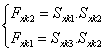(9)

The phase voltages vak, vbk, vck can be written as:(10)

With three possible output states for each of the three phases, there are a total of 27 possible switch combinations. The result of plotting each of the output voltages in a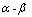reference frame is shown in Figure 2.

Figure 2 shows that the 27 switch combinations result in a total of 19 unique voltage vectors since some of the combinations produce the same voltage vector. These different combinations relate to different ways of connecting the load to the DC bus that result in the same voltage being applied to the motor.Figure 2. Space vector diagram showing switching states for a three-level NPC

The principle of the DTC control is based on stator flux and electromagnetic torque regulators using a bang–bang comparator with hysteresis. These regulators use the estimated errors of the control variables at each sampling time of operation. The considered controllers for the torque and the flux ensure the separate regulation of these two variables, as for the DC variations. When the level of torque or stator flux passes to the high or low hysteresis limit, a suitable voltage vector is applied to bring back each variable in its corresponding band.

The components of stator flux can be estimated by:(11)

The stator flux magnitude and its angle are given by:(12)

The electromagnetic torque can be estimated by: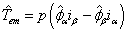(13)

The reference values of flux and torque are compared to their actual values and the resulting errors are fed into two hysteresis comparators.

The switching selection table for the conventional DTC for DSSM is given in Tables 2 and 3.The DTC block diagram of DSSM supplied by three-level NPC voltage source inverter in each star is represented by Figure 3.

DTC Based on Artificial Neural Networks Strategy

A general architecture graph of Multi-Layer Perceptron (MLP) is shown in figure 4. This network which can be multiplexed for each output of the controller has been found to possess acceptable performance in many industrial applications . The feed-forward topology shown in the network of figure 4 offers the advantage of simplicity and ease programming. Such a neural network contains three layers: input layer, hidden layers and output layer. Each layer is composed of several neurons. The number of the neurons in the output and layers depends on the number of the selected input and output variables. The number of hidden layers and the number of neurons in each depend on the system dynamic and the desired degree of accuracy.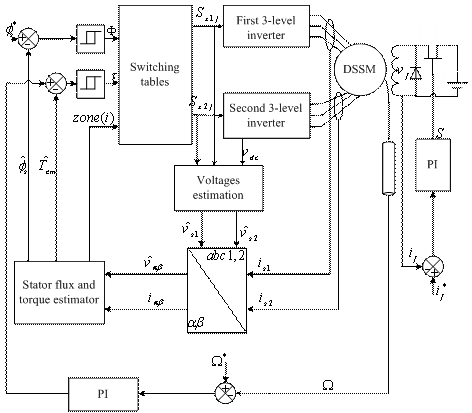Figure 3. Direct torque control scheme for DSSM (with j=1, 2, 3 or 4)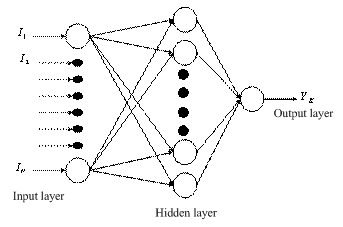Figure 4. Architecture of multilayer neural network

The structure of the neural network to perform the DTC applied to DSSM satisfactorily was a neural network with 3 linear input nodes, 12 neurons in the hidden layer, and 6 neurons in the output layer, as shown in Figure 5.Figure 5. Neural network structure for three-level DTC

The general structure of the double star synchronous machine with DTC-ANN using a three-level inverter in each star is represented by Figure 6. It includes closed loop control of speed using PI controller.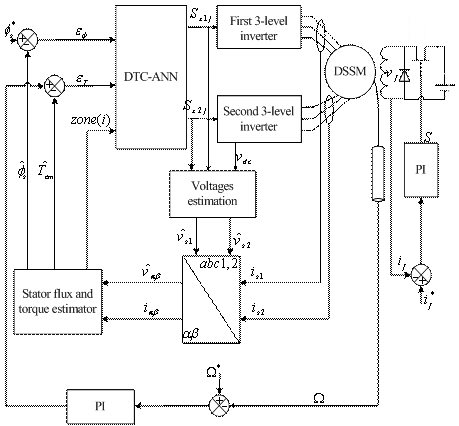Figure 6. Schematic diagram of artificial neural network based DTC for DSSM

Results and Discussion

The proposed control method has been tested under reversal speed condition whereas the machine is loaded at time 0s by a rated torque. The parameters of DSSM are given in the Appendix. The obtained three-level DTC-ANN results are presented and compared with those obtained by conventional three-level DTC. Figures 7 and 8 gather the whole of DTC results with its two variants above-mentioned.

The DSSM is accelerating from standstill to reference speed 100 rad/s. The system is started with full load torque (TL =11 N.m). Afterwards, a step variation on the load torque (TL =0 N.m) is applied at time t=1s. Afterwards it is decelerate to the inverse rated speed (-100 rad/s) at time t=1.5s.

Simulations results show that the system controlled by the proposed method is still little affected; it shows a good property of robustness in the face of external load disturbance and speed reversion.

The dynamic responses of mechanical speed, stator flux and electromagnetic torque are shown in figure 7 for conventional DTC and in figure 8 for DTC-ANN. The simulation results illustrate both the steady state and the transient performance of the proposed torque control scheme.

In figures 7 and 8, the speed response is closed to its reference without any overshoot and steady-state error and the flux is very similar to the nominal case while the electromagnetic torque returns to its reference value without an error. Thus decoupling between stator flux linkage and torque is ensured for the nominal load. It is important to notice in figure 8 that the stator flux ripples are considerably decreased.

The comparison between figure 7 and figure 8 reveals that the flux ripple of the proposed DTC-ANN is less than that of the conventional one. Furthermore, the obtained results show that the use of a three-level inverter instead of a two-level one improves the dynamical performance of the drive leads to lower ripples.

Also, it has been shown that using DTC-ANN, satisfactory dynamic is achieved. This can be manifested in fast torque response and robustness against load torque.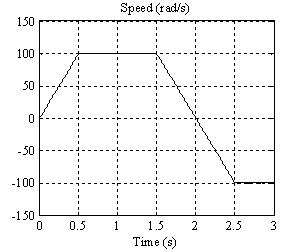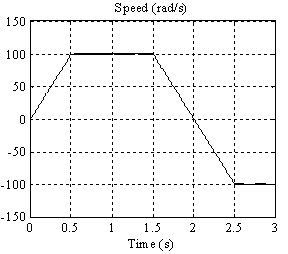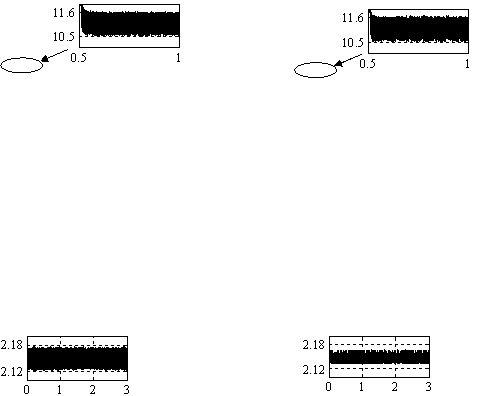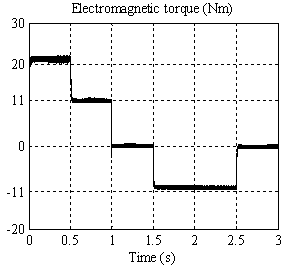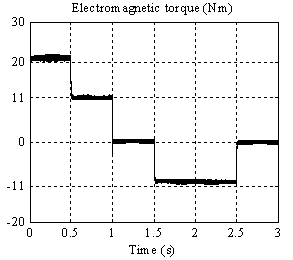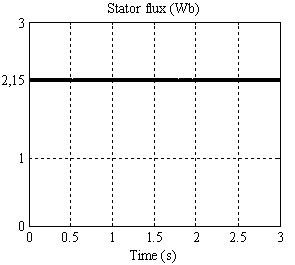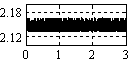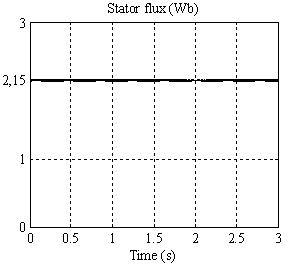Figure 7. Dynamic responses of DSSM controlled by DTC. Figure 8. Dynamic responses of DSSM controlled by DTC-ANN.

Conclusion

The presented approach provides a more accurate selection of the inverter state. Some simulation results were carried out to illustrate the effectiveness of the proposed control system. It is pointed out that the robustness of the controlled double star synchronous machine drive against speed and load torque variations is guaranteed. Furthermore, the proposed control scheme decreases considerably the stator flux ripples and assures good speed tracking without overshoot. The decoupling between the stator flux and the electromagnetic torque is maintained, confirming the good dynamic performances of the developed multiphase drive system.

Appendix

The rated values and parameters for the double star synchronous machine used in the simulation study are listed in Table 4.

Table 4. DSSM parameters

 Rated power 5 kW Rated phase stator voltage 232 V Rated stator frequency 50 Hz Pole pair number (p) 2 Stator resistance (Rs) 2.35 Ω Rotor resistance (Rf) 30.3 Ω d axis inductance (Ld) 0.3811H q axis inductance (Lq) 0.211H Rotor inductance (Lf) 15 H Mutual inductance (Mfd) 2.146 H Moment of inertia (J) 0.05Nms2/rad Friction coefficient (f) 0.001Nms/rad

References

1.            Kianinezhad R., Nahid B., Betin F., Capolino G., A novel Direct Torque Control (DTC) method for dual three phase induction motors, IEEE, 2006, p. 939-943.

2.            Benyoussef E., Meroufel A., Barkat S., Three-Level Direct Torque Control Based on Space Vector Modulation with Balancing Strategy of Double Star Synchronous Machine, Journal of Electrical Engineering, 2013.

3.            Tessarolo A., Giulivo D., Direct Torque Control of Induction Motors with Fuzzy Logic Controller, International Symposium on Power Electronics, Electrical Drives, Automation and Motion, 2010, p. 845-852.

4.            Yao W., Jinlong W., Xiao P., Zhang H., Study and Simulation of Space Vector PWM Control of Double-Star Permanent Magnet Synchronous Generator, IEEE 7th International Power Electronics and Motion Control Conference, 2012, p. 2448-2452.

5.            Kallio S., Karttunen J., Andriollo M., Peltoniemi P., Silventoinen P., Finite Element Based Phase-Variable Model in the Analysis of Double-Star Permanent Magnet Synchronous Machines, International Symposium on Power Electronics, Electrical Drives, Automation and Motion, 2012, p. 1462-1467.

6.            Kallio S., Andriollo M., Tortella A., Karttunen J., Decoupled d–q Model of Double Star Interior-Permanent-Magnet Synchronous Machines, IEEE Transactions on Industrial Electronics, 2013, 60(6), p. 2486-2494.

7.            Munduate A., Figueres E., Garcera G., Robust Model-Following Control of a Three-Level Neutral Point Clamped Shunt Active Filter in the Medium Voltage Range, International Journal Electric Power Energy System, 2009, 31(10), p. 577-88.

8.            Bhalodi H., Agarwal P., Space Vector Modulation with DC-Link Voltage Balancing Controlfor Three-Level Inverters, International Journal of Recent Trends in Engineering, May 2009, 1(3), p. 229-233.

9.            Kadri  F., Drid S., Djarah D. Djeffal F., Direct Torque Control of Induction Motor Fed by Three Phase PWM Inverter Using Fuzzy Logic and Neural Network, Sixth International Conference on Electrical Engineering, 2010, p. 12-16.

10.        Khodja  D., Boukhemis C., ANN Based Double Stator Asynchronous Machine Diagnosis Taking Torque Change into Account, International Symposium on Power Electronics, Electrical Drives, Automation and Motion, May 2010, p. 1125-1129.